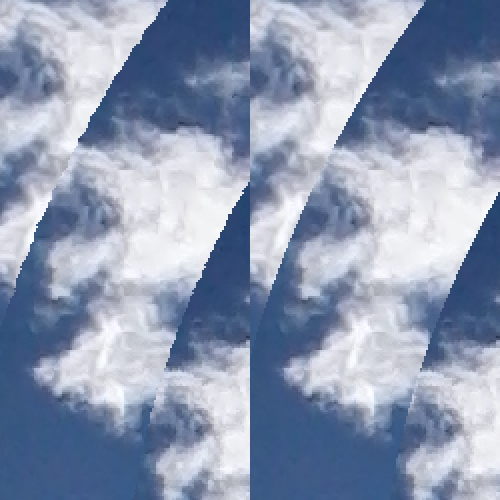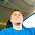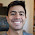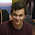## Friday, September 7, 2012

### The Fibonacci Vortex

Recently I came across this image called "Fibonnaci Vortex" by the artist Leif Podhajsky.

I thought it was pretty neat. I liked how the structure of the image - how it was created, mechanically - could be discerned just by looking at it. Yet the end result didn't look mechanical - it looked beautiful and infinite. I was inspired to write my own MATLAB code to perform this image manipulation. Here is some sample output:

With some post-processing and a better camera (the original image was from my cell phone), my image could be made to look much better.

The Code

At a glance, the code works like this:

1. Load the original image and store it as an x by y RGB matrix.

2. Find the first circular region - the outermost disc - in the matrix using the distance formula and store it as an RGB matrix. The area outside of the disc is zeroed out.
3. Repeatedly shrink and rotate this disc, each time overlaying it on the original image.

Coding Nuances

I ran into some coding difficulties that I ultimately solved in a slightly dubious way.

MATLAB conveniently provides the functions imrotate and imresize, but they do not work exactly as desired for this application.

Rotating a rectangular image by anything other than 180° or 360° inherently increases the rectangular envelope needed to fully contain the image. However, since the disc is centered and is wholly contained in the image, it will always stay within the image matrix. Therefore the dimensions of the disc's image matrix should stay the same size. This is achieved by specifying to imrotate to make the rotated image of the same dimensions as the original with the 'crop' parameter.

There's another problem though - during rotation, some areas outside the image are drawn into the frame. MATLAB assigns these new undefined regions to be pure black - RGB [0,0,0]. Ideally, these regions would be transparent, but that's not how the command works.

The imresize command works similarly. When an image is shrunk but its matrix dimensions stay the same, the image shrinks in the frame of its matrix and the new undefined regions are assigned to be pure black. Therefore it seemed convenient - for both rotation and resizing - to reserve the color pure black for transparent regions. However, I had to also account for the user inputting a dark image with patches of pure black.

I decided that in order to reserve pure blacks for transparency, I would have to eliminate them in the source image. Here is the function reserveBlacks that does this.

function R=reserveBlacks(R)
for a=1:3 % iterate through the color channels (RGB)
for yInd=1:yDim % iterate through the columns
for xInd=1:xDim % iterate through the rows
if R(yInd,xInd,a) == 0 % if pixel is black
R(yInd,xInd,a)=1; % make it off-black
end
end
end
end
end

If a pixel is pure black in any of its color channels, it is made off-black (i.e. a very dark gray - RGB [1,1,1]). This is not ideal, but it's also impossible to notice visually. And it greatly simplifies the image processing in MATLAB.

Setting the parameters

In my code, a disc is defined by its scale and angle of rotation, both relative to the original disc. It may seem logical to derive each disc from the one preceding it, but the problem is that rotating and resizing images is lossy, and iteratively repeating this lossy process would result in the discs looking more distorted with each successive level.

In the original image, the rate of change in scale between discs is not constant. It decreases as the discs get smaller, as if asymptotically. Therefore I thought to somehow use the function y=1/x, which decreases to asymptotically approach 0 (below).

The angle change between discs appeared to be roughly constant. Therefore I set up these equations, to be updated for each disc.

scale=scale-1/(level+A)*B; % level is the current disc number
angle=angle+C;

The variable level keeps track of the current disc number and increases as the discs get smaller. The variables AB, and C were placeholders for parameters, to be figured out later.

Choosing the disc parameters

Because performing the entire image manipulation process on an image took a minute or two, I wrote another program to quickly show an approximation of the final image. This would enable me to quickly figure out the correct parameters to govern scale and angle so that the image would look right.

Instead of performing the image manipulations, the program draws circles where the discs' edges will be in yellow and shows their angle in green. Here is an example using another picture.

Using this program, I arrived at the constants A=1.5, B=0.42, and C=10. The constant A shifts the 1/x function to the left by 1.5 units to get rid of its steepest part, which made the discs looks proportionally wrong. The factor of 0.42 was included to scale the overall effect of the 1/x function.

Final touches

To make a new disc, my program rotates and then scales the original disc. The problem is that both of these operations are lossy, so after both have been completed, the crisp circular border of the disc becomes distorted. To fix this, I used the distance formula to crop a circular region slightly smaller than the disc, getting rid of imperfections in its border.(detail) Before and after edge cleaning.

Gallery

Here are some more images I made using my program, all of which use approximately similar values for
AB, and C.

1.2.1.